### Triangulation Algorithm

BACKGROUND

Polygon triangulation is an essential problem in computational geometry because working with a set of triangles is faster than working with an entire polygon in case of complex graphics.

Polygons are very convenient for computer representation of real world object boundaries. Real world objects can be very complex as they can include polygons with a few thousand vertices, they may be convex or concave, have self intersections and may include nested holes and often there is need to decompose the polygons into simpler components that can be easily and rapidly processed.

Several algorithms such as color filling, character recognition, shading and shortest path rely entirely upon partitioned polygons.

A video card, also referred to as a graphics accelerator card / display adapter is an item of personal computer hardware whose function is to generate and output images to a display. The graphics card is responsible for simplification (Triangulation) of complicated display objects in real time so that the main processor can handle the display objects much faster. Without the simplification the display will not be processed in real time and the display will be delayed. For example in computer games, the display depends on the user inputs, like if one is playing a car race game and if the user presses ↑ key, the car should move forward immediately; but if the frames or the display objects are not processed fast enough then the display is rendered after some delay i.e. the perception of car moving forward is delayed. This delay hampers the real time game play experience. Triangulation is one of the many logics which are present in a graphics card which helps in accelerating the rendering of complex objects on computer screen.

The problem of polygon triangulation can be stated as “given the co-ordinates of the n vertices of a polygon P in order around P, find n-3 diagonals that partition P into n-2 triangles”.

See figure 1. Red lines represent actual polygon contour whereas inner black lines represent new diagonal edges introduced after triangulation of a polygon.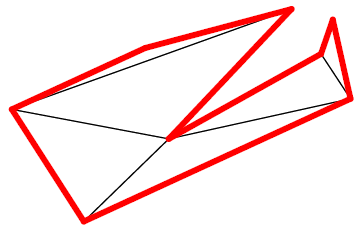Figure 1 - A triangulated polygon

SEIDEL'S ALGORITHM

Given the importance of triangulation, a lot of effort has been put into finding a fast polygon triangulating routine. The simplest recursive triangulation of a polygon runs in time O (n3) by cutting ears from the polygon. O (n2) algorithms have been known since at least 1911. But it wasn’t until 1978, when Garey et al found an O (n log n) algorithm that real work started in this field. A variety of new algorithms were found pushing the limit further and further down. In 1984 it was shown that given a trapezoidation of a polygon, a triangulation could be obtained in linear time and further work focused on finding an efficient way of computing such a trapezoidation. However, the outstanding open problem in computational geometry, that of the lower time bound of triangulation, was not solved until Chazelle found a purely linear algorithm in 1990. This algorithm is very complex to implement.

In 1991, Seidel found a practical algorithm for triangulating polygons with an expected running time of O (n log*n). The algorithm can triangulate any set of overlapping and self-intersecting polygons and lines in the plane with near-linear expected time. Its virtues lie in its simplicity. It uses no divide-and-conquer or recursion and no “Jordan Sorting”. Its expected performance admits a very straightforward and self-contained analysis. Finally, it is practical and relatively simple to implement, a property that very few, if any, of the algorithms mentioned can claim.

It should also be mentioned that in 2000 Amato et al. found a similar linear algorithm with far less overhead than Chazelle’s, by using randomization as in Seidel’s algorithm. This would still be a formidable job to implement with all its details.

Seidel’s algorithm suggests following steps for triangulation of a polygon: -
1. Trapezoidal decomposition or trapezoidation
2. Generation of monotone mountains
3. Triangulation by ear clipping method

1. Trapezoidal decomposition or trapezoidation

A trapezoidation is formed by extending a horizontal ray in each direction from every vertex, and stopping the ray as soon as it hits another edge. This will divide the plane into regions by edges and rays, and each such region is a trapezoid. A trapezoid has a horizontal upper and lower boundary, and an edge or a piece of an edge as a boundary on either side. Either the upper or lower boundary may have zero length, in which case the trapezoid will be a triangle. The set of trapezoids obtained in this manner is called a trapezoidation, and is unique for any given polygon. See figure 2.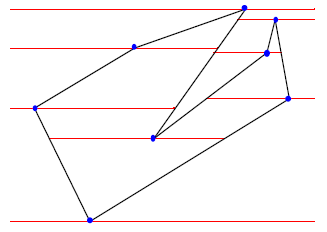Figure 2 Trapezoidal decomposition

Seidel’s algorithm is a randomized way of computing trapezoidation. This means that every edge is equally likely to be added in the trapezoidation. For this purpose a random sequence of edges is generated. This ensures that actual running time will not be significantly worse than expected running time.

Trapezoidation routine starts with an empty plane and adds in edges one by one. The algorithm checks if endpoints of the edge are added to the trapezoidation already. Those which have not been added are added one by one. This will divide the region (in which point is added) into two by means of horizontal rays starting from that point and extending in both directions (left & right). Next the edge is added between two points. This is done by starting from the top point and moving down one region at a time, until bottom point is reached. For each region traversed in this manner, the region is divided in two by means of the new edge. Whenever this causes two regions on top of each other to have same left and right hand boundary, these two regions are merged into one. Each of the regions created in this manner will be a trapezoid. Note that in doing this; we never need to explicitly calculate the intersections of edges and rays.

Each trapezoid will know which edges and rays it is bounded by. For this we will have a special memory structure which stores information about each trapezoid. This structure is explained as below.

Trapezoid Data Structure:-
High - Upper vertex of the trapezoid
Low - Lower vertex of the trapezoid
U1, U2 - Upto two adjacent trapezoids above
D1, D2 - Upto two adjacent trapezoids below
U3 - Third extra region above (if any)
U3 SIDE - Determines whether the third extra region is towards left or right
LSEG, RSEG - Left and right edges of the trapezoid
SINK - Position of trapezoid in tree structure (discussed later)
STATE - Represents validity of trapezoid (Inside or outside)

The algorithm also needs to be able to efficiently tell where to place new points in the current trapezoidation. One way of doing this is by creating a tree lookup structure for trapezoids. The tree starts only with a root representing the original empty plane and each time a region is split in two, the corresponding leaf in the tree is changed into a node with two children. This new node can either be a vertex or an edge. The two children represent two resulting trapezoids. Thus each leaf in the tree represents a trapezoid (region) and each node in the tree represents either vertex or edge. By querying whether the point we are adding is above or below a vertex in the tree, or to which side of an edge it is, we can traverse the tree to find the correct region in which the point will be added. The tree is a strict binary tree and also called as “query structure”. This tree structure is explained below.

Query Structure:-
Node type - Whether the node in tree is vertex or edge or trapezoid
Segment number - Points to vertex or edge if node type is either vertex or edge
Trapezoid number - Points to trapezoid in trapezoid data structure if node type is trapezoid.
Left - Points to left child node
Right - Points to right child node
Parent - Points to parent node

Steps for adding an edge in the trapezoidation are as follows:-
1. Select an edge from randomly generated sequence of edges

2. Find higher vertex of that edge

3. Traverse the tree to find correct region in which higher vertex will be added
If vertex is not already added, change the leaf node (trapezoid) into a vertex node. This node will have two trapezoids as its left and right children. Left child represents region below the vertex whereas right child represents region above the vertex. Right child trapezoid is old region to be split by the vertex. Left child trapezoid is new region introduced after addition of vertex. Update the trapezoid structure.
If vertex is already added (as part of previously added edge), just traverse the tree to find the region in which the vertex reside. This is required for addition of an edge.

4. Add lower vertex to the trapezoidation and follow the same procedure as for higher vertex to traverse the tree and find correct region.

5. Finally, add the edge starting from higher vertex to lower vertex. This edge will split all the regions which are below higher vertex and above lower vertex. Update the trapezoid structure, for regions split by the edge.

Let us take an example to understand the procedure

Consider trapezoidation of polygon as in figure 3.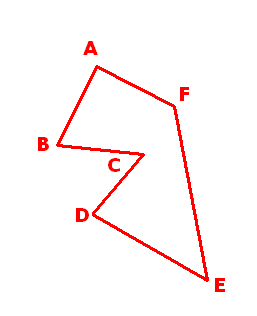Figure 3 - Sample Polygon

Let edge CD is added to the trapezoidation. Note that Query structure will contain only one region before addition of edge CD as CD is the first edge to be added.

Add point C to trapezoidation as it is higher vertex.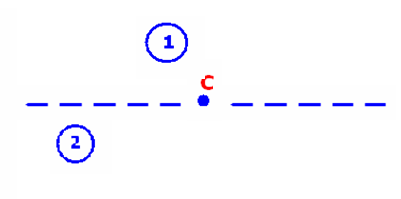Update tree structure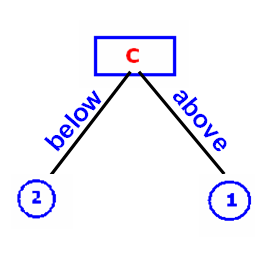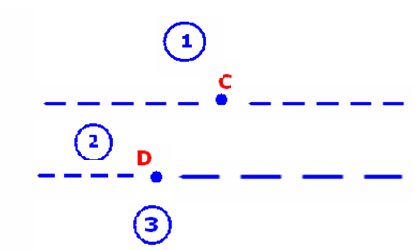Traverse the tree to find correct region. Point D will split region 2.
Update the tree structure by adding point D and introducing two leaf nodes to the left and right of D. Left node represents region 2 whereas right node represents new region viz. region 3.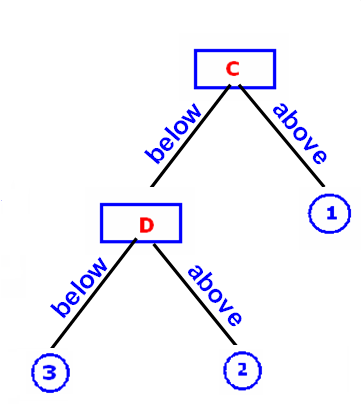Add edge starting from point C to point D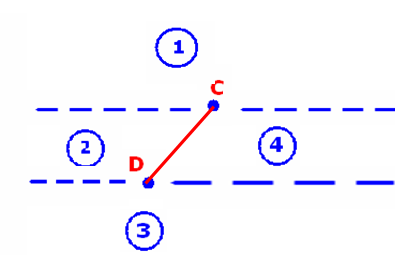Edge CD will split region 2. Update tree structure by creating new node representing
edge CD. Left child of node CD will be region 2 (old region) and right child will be region 4 (new region).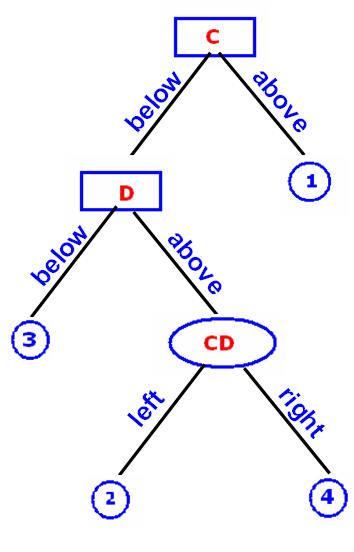Follow the same procedure for remaining edges.

Note that, for node type vertex in the tree, left of represents below where as right of represents above. For node type edge left of represents left and right of represents right.

While adding the edge to trapezoidation, trapezoid structure must be updated also.

Different condition that can occur while adding part of an edge in a region are explained below. These conditions are discovered using extensive trial and error method and lexicographic techniques.

Red lines represent already inserted edges. Blue line represents current edge being added.

Condition 1: No trapezoids below (This condition is not possible)

Condition 2: Only one trapezoid below

Sub condition 1: Two trapezoids above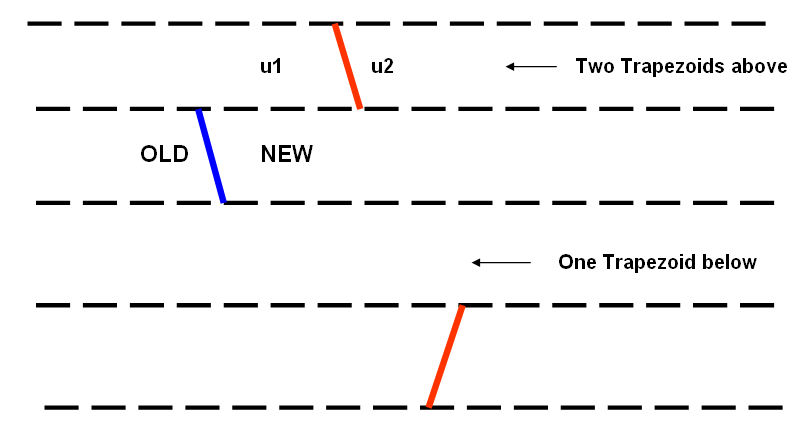Sub condition 2: Three trapezoids above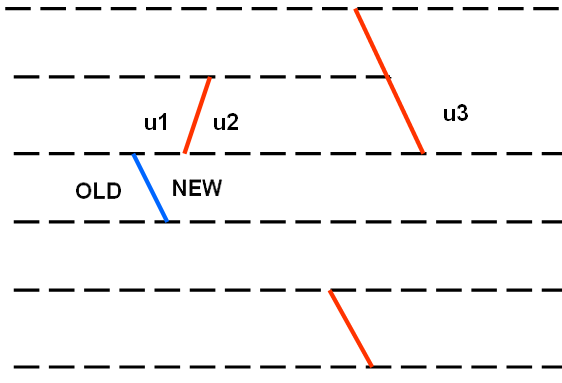Sub condition 3: Only one region above and left to right upward cusp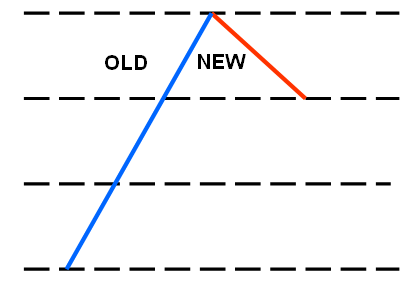Sub condition 4: Only one region above and right to left upward cusp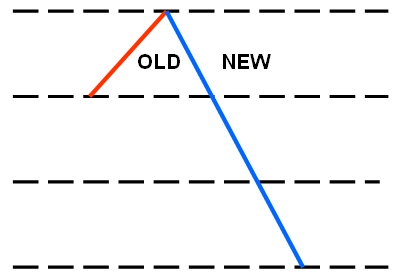Sub condition 5: Fresh segment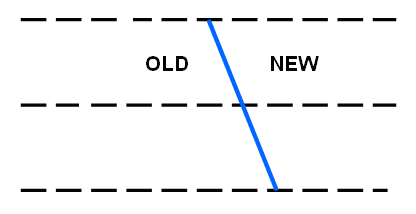Sub condition 6: Last region forms left to right downward cusp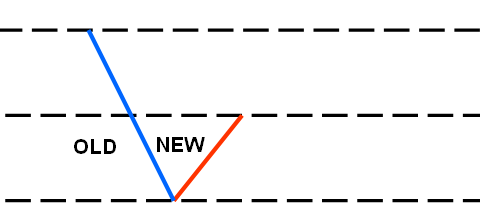Sub condition 7: Last region forms right to left downward cusp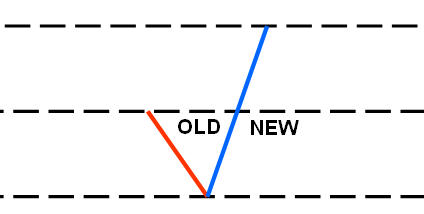Sub condition 8: Intermediate region (Addition of edge results in three upper neighbors)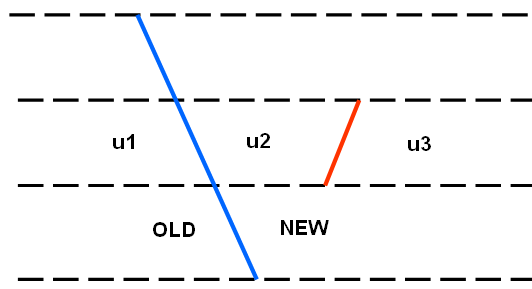Condition 3: Two trapezoids below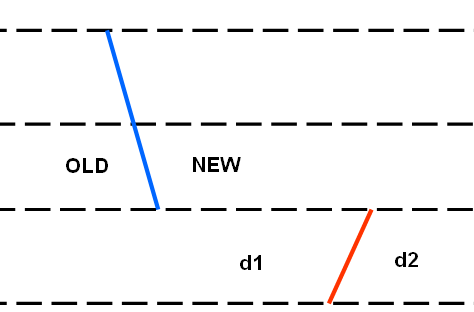Sub conditions handled in this part are same as those in condition 2.
Only exception is that we must check whether the edge being added divides d1 or d2.

2. GENERATION OF MONOTONE MOUNTAINS

Given the trapezoidation of a polygon, a triangulation can be easily computed in linear time. Let us consider only those trapezoids that are inside the polygon, as these are the ones we will want to triangulate. Trapezoids which lie inside the polygon are found out using fill rule. Fill rule and it’s realization for triangulation is explained in section later below.

First of all, note that each trapezoid will be empty, i.e. there will be no vertices or edges inside it. Further note that the upper and lower boundaries of each trapezoid are created by the extension of rays from vertices, such that each trapezoid has one bounding upper and one bounding lower vertex. Lower is defined here lexicographically, i.e. of two vertices with the same y-coordinate; the rightmost will be considered lower. This is assumed for all vertices and it assures that no edge is being treated as if it was horizontal, and that no trapezoid will have more than one upper and one lower bounding vertex. In some cases the two bounding vertices will be on the same side of the trapezoid, and in other they may be on opposite sides, or in the middle. Whenever the two vertices are not along the same edge, one can draw a diagonal between them without intersecting any edges since each trapezoid is empty. Addition of these diagonals is illustrated in figure 4. Resulting polygons bounded by edges and diagonals are termed as monotone mountains.

Monotone mountains have one edge called the base, which extends from the uppermost point to the lowermost point. The rest of the vertices form what is called a monotone chain, which is characterized by the fact that traversing polygon from the top, every vertex will be lower than the previous one. If a monotone mountain is put with its base extending horizontally, it resembles a mountain range, hence its name. See figure 4.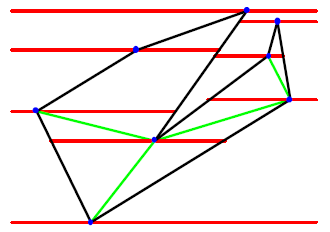Figure 4 - Introduction of trapezoid diagonals

Fill Rule

A simple, non-self-intersecting closed path divides the plane into two regions, a bounded inside region and an unbounded outside region. Note that knowing the orientation of the outermost path (i.e. clockwise or anticlockwise) is not necessary to differentiate between the inside and outside regions.

A path that self intersects, or that has multiple overlapping sub paths, requires additional information in order to define the inside region. Two rules that provide different definitions for the area enclosed by such paths, known as the non-zero and even/odd fill rules are discussed in this paper.

To determine whether any point in the plane is contained in the inside region, imagine drawing a line from that point out to infinity in any direction such that the line does not cross any vertex of the path. For each edge that is crossed by the line, add 1 to the counter if the edge starts from top to bottom (starting point is above) and subtract 1 if the edge starts from bottom to top (starting point is below). In this way, each region of the plane will receive an integer value.

The non-zero fill rule says that the point is inside the polygon if the resulting sum is not equal to zero.

The even/odd fill rule says that the point is inside the polygon if the resulting sum is odd, regardless of sign (i.e. -3 is odd).

Consider the star-shaped path shown in Figure 5 and 6 below, indicated with solid lines. The orientation of the lines making up the path is indicated with arrows. An imaginary line to infinity starting in the central region of the star is shown as a dashed line pointing to the right. Two edges of the star cross the line to infinity going top to bottom, indicated by the downward-pointing arrows. The central region therefore has a count of +2. According to the even/odd rule, it is outside the path, whereas according to the non-zero rule it is inside.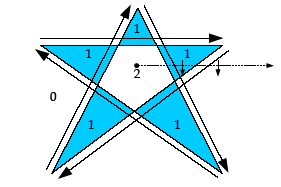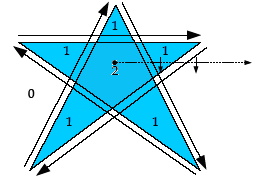Figure 5 - Even/odd fill rule         Figure 6 - Non-zero fill rule

Fill Rule and Triangulation

Trapezoidation is the most important step in Seidel’s algorithm. But as we know that, all trapezoids formed in this step do not lie inside the polygon. Some of them lie outside or inside the hole. So before going to build monotone chains, its necessary to mark all trapezoids as inside or outside. Only those trapezoids which lie inside the polygon are given as input to next step.

The question is how we can mark trapezoids as inside or outside without knowing the orientation of the sub paths (i.e. clockwise or anti-clockwise). At this stage fill rule comes in picture. With the help of fill rule and query structure built in trapezoidation phase we can easily determine whether the trapezoid is inside or outside. Note that interior seed point is not at all required.

As we know that each interior trapezoid is bounded by edges to its left and right and for any trapezoid we can determine its position in the query structure (remember sink?). Once the trapezoid is located in tree structure, we traverse upwards until the node representing left or right edge of that trapezoid is encountered. If left edge is first encountered our direction will be left and if right edge is first encountered our direction will be right. This is done only for first trapezoid to determine direction of infinity line. From this node (left or right edge) traverse the tree downward to left or right (depending on direction) until node representing a trapezoid is reached. This trapezoid is adjacent to the trapezoid with which we started with. The nodes of type vertex also need to be handled. For this additional variable to indicate the sub direction is required. Initially we set this sub direction to left. So while traversing downwards for odd occurrence of vertex node (1st, 3rd…) we go to left and change the sub direction to right. For even occurrence of vertex node (2nd, 4th) we go to right and change the sub direction to left. This alternate switching of sub direction is related to the property of the tree structure i.e. while adding the edge to the tree structure higher point is added first and then lower point is added. While traversing downwards if node of type edge is encountered and if direction is left (not sub direction) we go to right otherwise (direction is right) we go to left. If a node representing trapezoid is reached, we are in the region adjacent to the region in which we were previously in.
For this newly encountered trapezoid check if it has both left and right segments or not. If it has, traverse the tree upward until the required segment node is not reached.
If direction is left we must traverse up to the node representing left edge of this trapezoid and if direction is right we must traverse up to the node representing right edge of this trapezoid. Once the required edge is reached, again traverse downward (depending on direction) until trapezoid node is reached. If the trapezoid which does not have either left or right segment is reached, it means that we are now outside the polygon and all the edges between the path of infinity line are crossed. For each such edge crossed, we either increment or decrement the counter depending on the direction of edge (upward or downward).

If the counter is non-zero, according to non-zero fill rule, corresponding trapezoid is inside.

If the counter is non-zero and odd, according to even/odd fill rule, corresponding trapezoid is inside.

We continue with above procedure unless we are outside the given input polygon. This is explained with suitable example as below.

Consider following input polygon.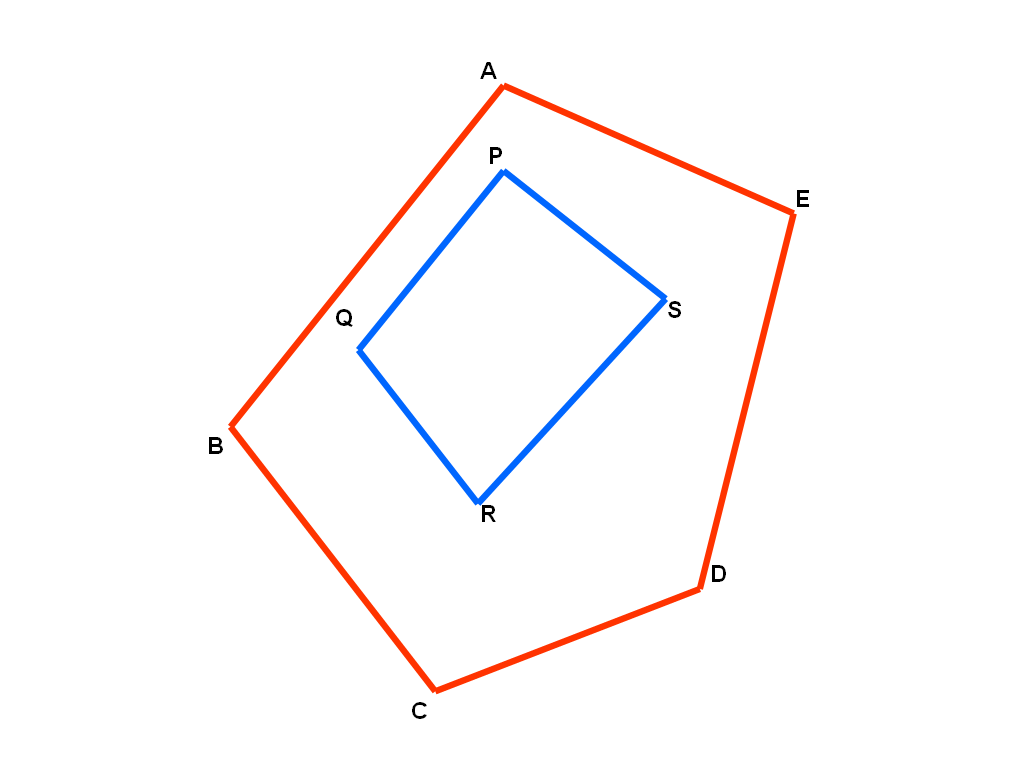Trapezoidation of above polygon is as shown below.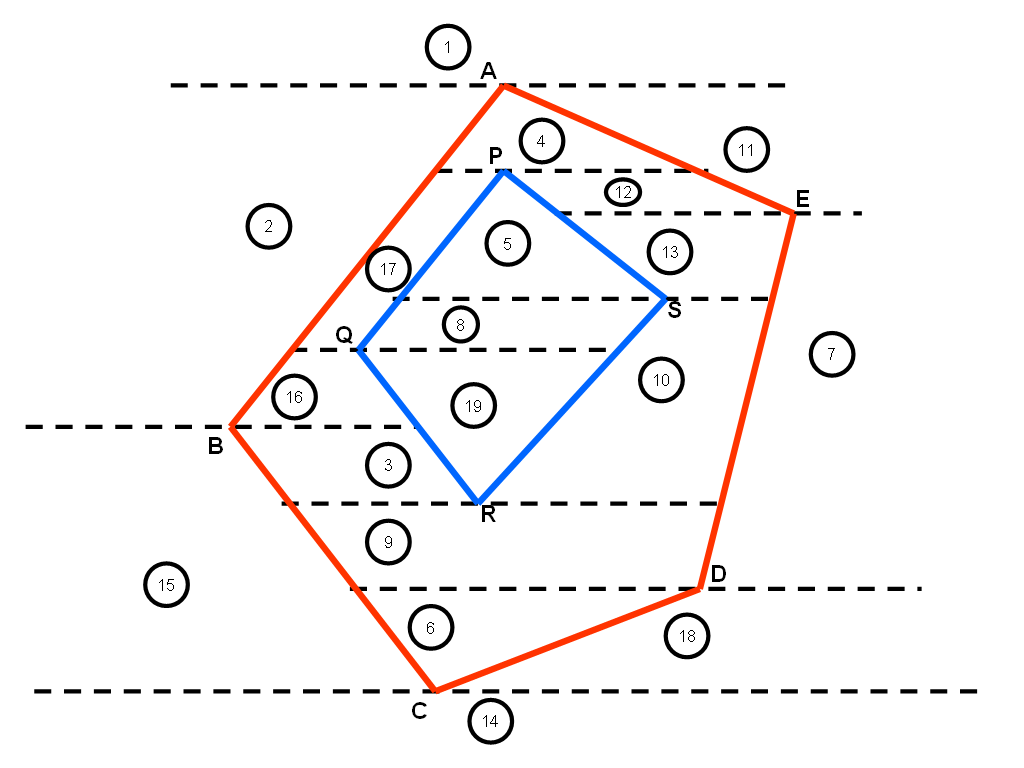Query structure for above trapezoidation is as shown below.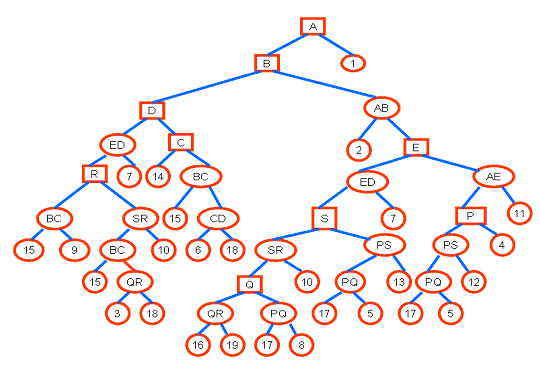Now suppose we want to determine whether trapezoid 10 is inside or outside the polygon.
1. Traverse the tree upward until left or right edge of trapezoid 10 is reached.
2. In this case edge SR i.e. left edge will be reached first. Decrement counter, as edge SR is going upward.
3. From now onwards our direction will be left.
4. From edge SR traverse to the left until adjacent trapezoid is reached.
5. In our case, node Q in encountered (vertex node). This is first occurrence (odd) of vertex node so we proceed to left.
6. Edge QR is encountered. As the direction is left we go to right from this node.
7. Trapezoid 19 is reached which is adjacent to trapezoid 10.
8. Trapezoid 19 has both left as well as right segments.
9. From trapezoid 19, traverse upward until left segment of 19 i.e. QR is reached (note our direction is now left).
10. Increment the counter as edge QR is going downward.
11. Again from edge QR, traverse downward to the left (our direction is left) until trapezoid node is reached. Here trapezoid 16 will be encountered.
12. Trapezoid 16 has left as well as right segment.
13. Again from trapezoid 16, traverse upward until left segment of 16 i.e. AB is reached.
14. Increment the counter as edge AB is going downward.
15. From edge AB, traverse downward to the left (our direction is left) until trapezoid node is reached. Here trapezoid 2 will be encountered.
16. Trapezoid 2 does not have left segment, which means we are outside the polygon.
17. Check the counter value. For our case counter=1. According to non-zero as well as even/odd fill rule region 10 is inside the polygon.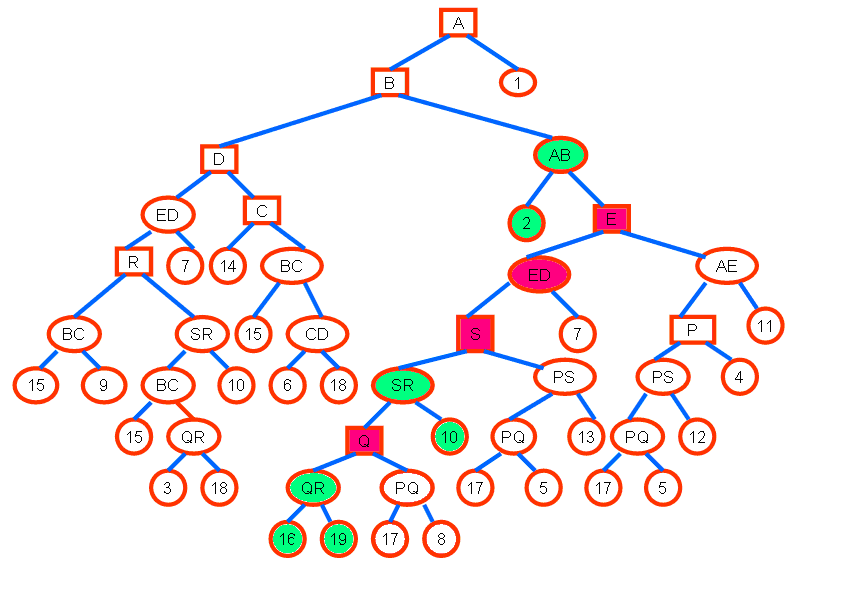3. TRIANGULATION BY EAR CLIPPING METHOD

If the inside angle between two edges of the polygon is less than л, the vertex is convex. If the triangle described by a convex vertex and its two neighbors contain no other vertices, the triangle is an ear of the polygon. Any convex vertex of the monotone chain is the tip of an ear, with the possible exception of the vertices of the base. Cutting such an ear off from a monotone mountain will always result in a smaller monotone mountain. This leads to a simple linear procedure for triangulating such polygons. Start with a list of all these convex vertices, and run through the list, cutting off each convex vertex and creating a triangle. For each vertex that is being cut, if its neighbors were not already convex, see if they have now become convex, and if either one has, add it to the end of the list. Once you have reached the end of the list, you are done. The algorithm is summarized in Table1.

 Monotone Mountain Triangulation Initialize an empty list Add all convex vertices, excluding the endpoints of the base to the list While list is not empty Cut off the corresponding ear of the monotone mountain Delete the vertex from the list For the two neighbors of the vertex If the neighboring vertex is not an endpoint of the base, and was made convex by cutting off the ear, then add this neighbor to the list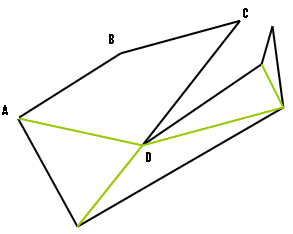Figure 7 - Monotone Chain             Figure 8 - Triangulated Polygon

After trapezoidation and generation of monotone mountains as you see in the Figure 7 A-B-C-D is the only partition that is not yet triangulated. So the triangulation is completed using ear clipping. B is a convex vertex as angle A-B-C is less than л and there is no vertex between A and C so diagonal is added between A and C and we get the final triangulated output as shown in Figure 8.

Some sample outputs from 'C++' implementation of this algorithm:-

Simple Polygon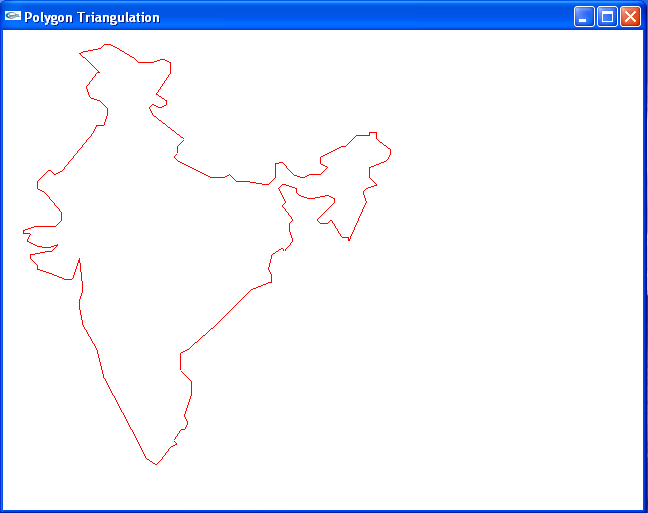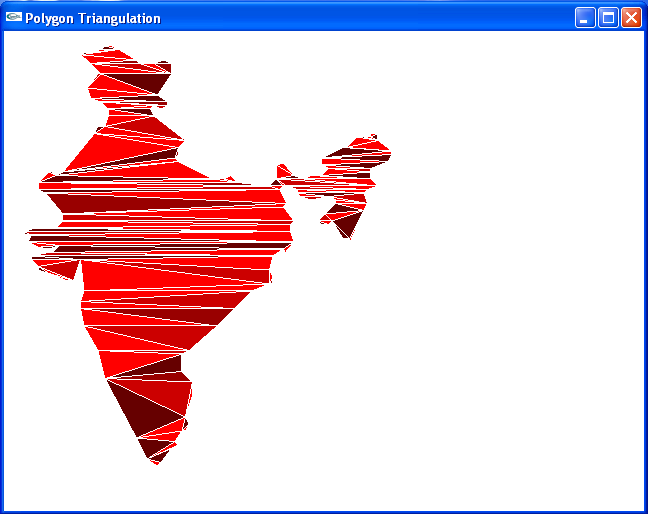Polygon with Holes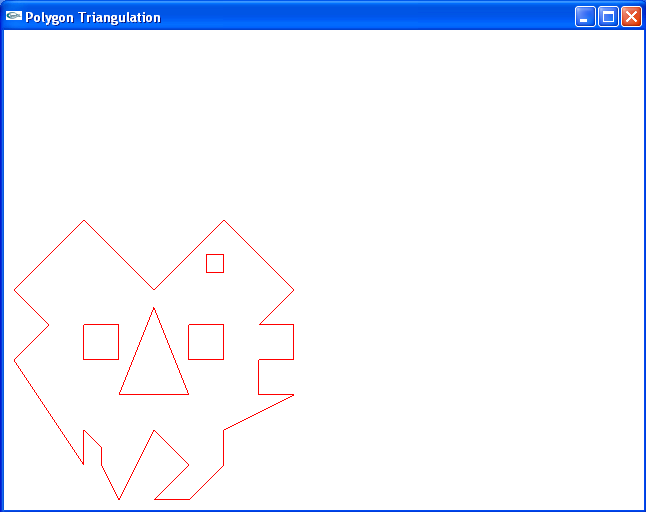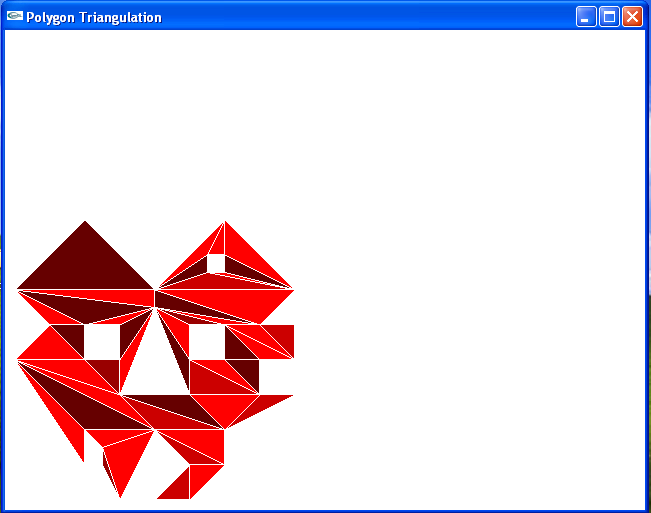Concentric Polygons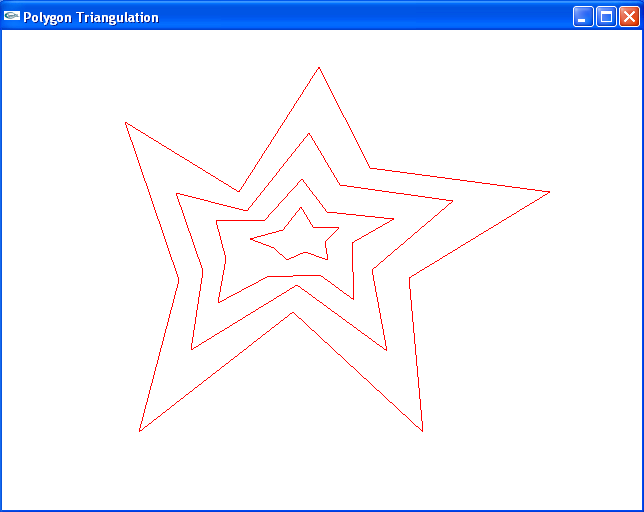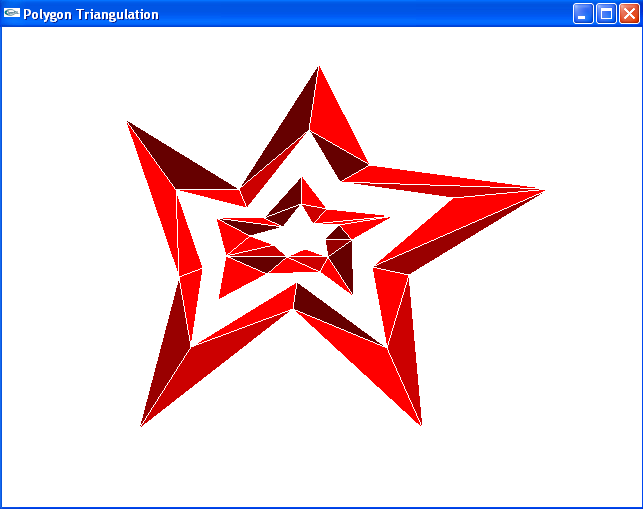Polygons with Multiple Holes at Various Depths of concentricity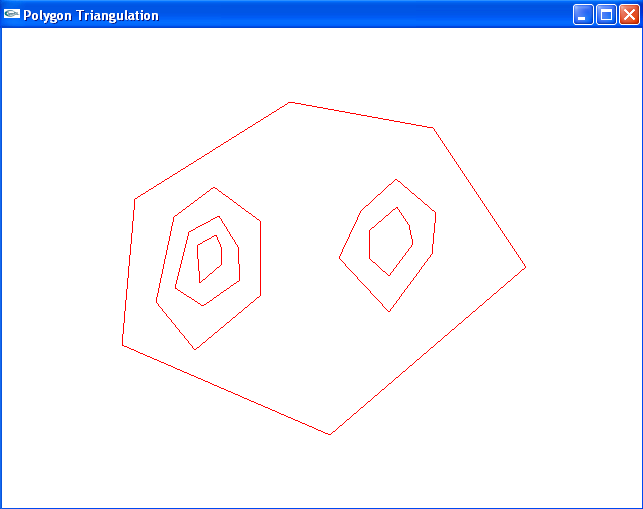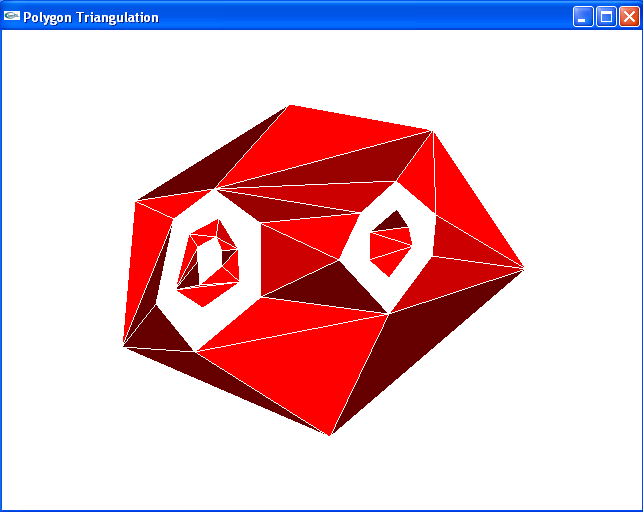Multiple Polygons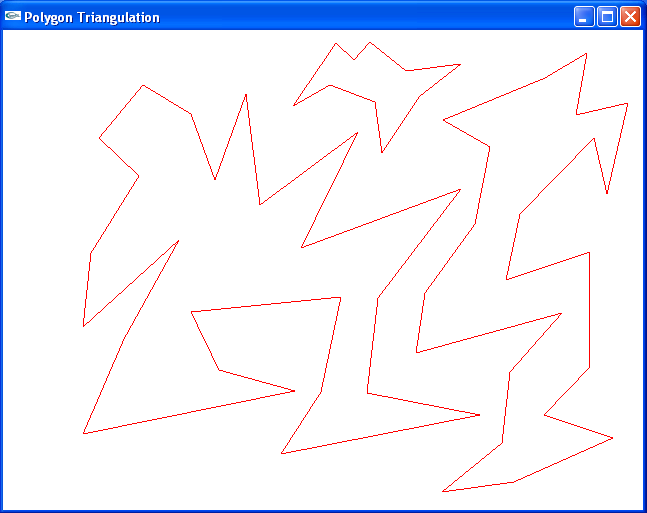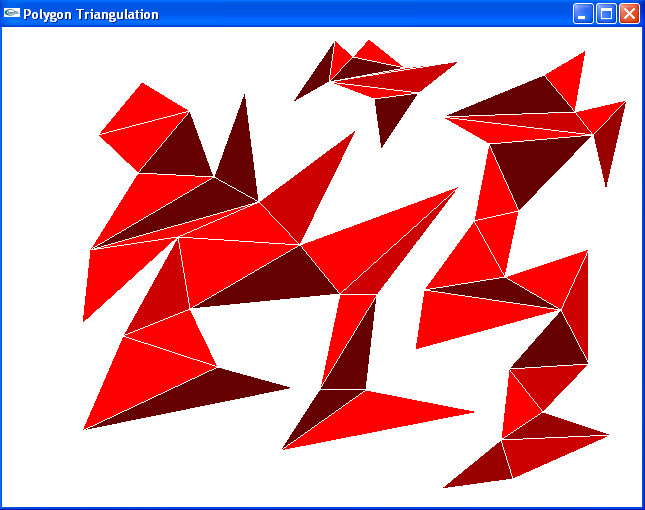A small demo video showing different polygon triangulation outputs obtained from implementation of above algorithm -